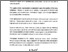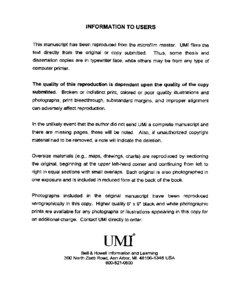Title:

# Phase transitions in superionic PbSnF₄

Madamba, Maria Cecilia S (1998) Phase transitions in superionic PbSnF₄. Masters thesis, Concordia University.Preview Text (application/pdf) MQ39072.pdf 6MB

## Abstract

PbSnF$\sb4$ is the highest performance fluoride ion conductor known to date. We have studied the phase transitions it undergoes under three different conditions: (i) versus the amount of hydrofluoric acid used for the preparation by the aqueous route, (ii) upon application of mechanical energy, and (iii) versus temperature. The addition of an aqueous solution of lead(II) nitrate to a fresh aqueous solution of SnF$\sb2$ results in the precipitation of $\alpha$-PbSnF$\rm\sb4(aq\sb1$), which is very highly strained in the ($\vec a,\vec b$) plane of the tetragonal unit-cell. If a very minor amount of hydrofluoric acid is used, the strain increases and o-PbSnF$\sb4$ is obtained. The $\alpha$-PbSnF$\sb4\to$ o-$\rm PbSnF\sb4$ transition is mostly a bidimensional phase transition, which includes a highly disordered intermediate "transitional phase". Ball milling results in a phase transition, giving microcrystalline disordered $\mu\gamma$-PbSnF$\sb4$, a cubic $\beta$-PbF$\sb2$ like phase. Tests were performed to make sure the $\mu\gamma$-PbSnF$\sb4$ samples are indeed microcrystalline cubic PbSnF$\sb4$ and not a mixture of microcrystalline $\beta$-PbF$\sb2$ and amorphous SnF$\sb2$. Milling $\alpha$- and $\beta$-PbF$\sb2$ for comparison and testing showed that both $\beta\to\alpha$ and $\alpha\to\beta$ transitions take place upon milling. Stirring $\alpha$-PbF$\sb2$ in an aqueous solution of SnF$\sb2$ results in the formation of $\rm\alpha$-PbSnF$\rm\sb4(aq\sb2)$ or $\rm Pb\sb2SnF\sb6$ depending on the conditions whereas no reaction occurs with $\beta$-PbF$\sb2$. When all the phases of PbSnF$\sb4$ are heated, phase changes take place versus temperature and time. Our findings provide key knowledge that will have to be taken into account in the fabrication of practical devices using PbSnF$\sb4$ for guaranteed reproducible results, long term stability and stable output of the device.

Divisions: Concordia University > Faculty of Arts and Science > Chemistry and Biochemistry Thesis (Masters) Madamba, Maria Cecilia S xix, 195 leaves : ill. ; 29 cm. Concordia University M.Sc. Chemistry 1998 Denes, Georges QD 561 M32 1998 664 Concordia University Library 27 Aug 2009 17:13 13 Jul 2020 19:47 https://concordiauniversity.on.worldcat....
All items in Spectrum are protected by copyright, with all rights reserved. The use of items is governed by Spectrum's terms of access.

Repository Staff Only: item control pageResearch related to the current document (at the CORE website)
Back to top